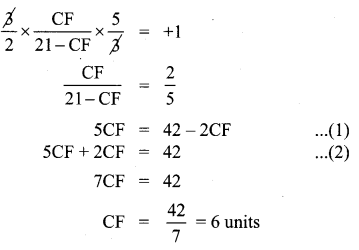## Tamilnadu Samacheer Kalvi 10th Maths Solutions Chapter 4 Geometry Unit Exercise 4

Question 1.
In the figure, if BD⊥AC and CE ∠ AB, prove thatSolution:
In the figure’s ∆AEC and ∆ADB.
We have ∠AEC =∠ADB = 90 (∵ CE ∠AB and BD ∠AC)
and ∠EAC =∠DAB
[Each equal to ∠A]
Therefore by AA-criterion of similarity, we have ∆AEC ~ ∆ADB
(ii) We have
∆AEC ~ ∆ADB [As proved above]
⇒ $$\frac{C A}{B A}=\frac{E C}{D B} \Rightarrow \frac{C A}{A B}=\frac{C E}{D B}$$
Hence proved.Question 2.
In the given figure AB||CD || EF . If AB = 6 cm, CD = x cm, EF = 4 cm, BD = 5 cm and DE = y cm. Find x and y.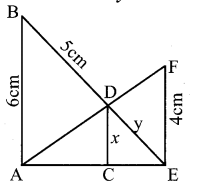Solution:
In the given figure, ∆AEF, and ∆ACD are similar ∆s.
∠AEF = ∠ACD = 90°
∠A = ∠A (common)
∴ ∆AEF ~ ∆ACD (By AA criterion of similarity)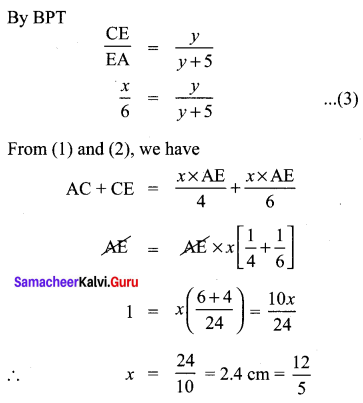Subtstituting x = 2.4 cm in (3)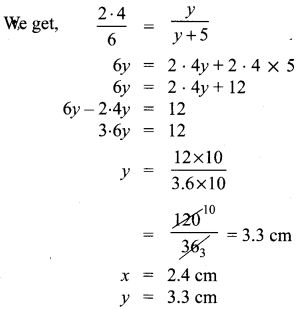Question 3.
O is any point inside a triangle ABC. The bisector of ∠AOB , ∠BOC and ∠COA meet the sides AB, BC and CA in point D, E and F respectively.
Show that AD × BE × CF = DB × EC × FA
Solution:
In ∆AOB, OD is the bisector of ∠AOB.In ∆BOC, OE is the bisector of ∠BOC
∴ $$\frac{\mathrm{OB}}{\mathrm{OC}}=\frac{\mathrm{BE}}{\mathrm{EC}}$$ …………. (2)
In ∆COA, OF is the bisector of ∠COA.
∴ $$\frac{\mathrm{OC}}{\mathrm{OA}}=\frac{\mathrm{CF}}{\mathrm{FA}}$$ …………… (3)
Multiplying the corresponding sides of (1), (2) and (3), we get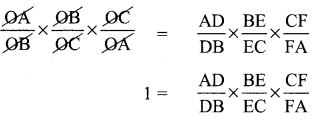⇒ DB × EC × FA = AD × BE × CF
Hence proved.

Question 4.
In the figure, ABC is a triangle in which AB = AC . Points D and E are points on the side AB and AC respectively such that AD = AE . Show that the points B, C, E and D lie on a same circle.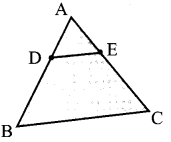Solution:
In order to prove that the points B, C, E and D are concyclic, it is sufficient to show that ∠ABC + ∠CED = 180° and ∠ACB + ∠BDE = 180°.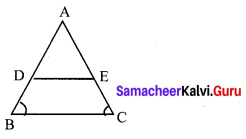In ∆ABC, we have AB = AC and AD = AE.
⇒ AB – AD = AC – AE
⇒ DB = EC
Thus we have AD = AE and DB = EC. (By the converse of Thale’s theorem)
⇒ $$\frac{\mathrm{AD}}{\mathrm{DB}}=\frac{\mathrm{AE}}{\mathrm{EC}}$$ ⇒ DE||BC
⇒ ∠ABC + ∠BDE = ∠ADE + ∠BDE (Adding ∠BDE on both sides)
⇒ ∠ABC + ∠BDE = 180°
⇒ ∠ACB + ∠BDE = 180° (∵ AB = AC ∴ ∠ABC = ∠ACB)
Again DE || BC
⇒ ∠ACB = ∠AED
⇒ ∠ACB + ∠CED = ∠AED + ∠CED (Adding ∠CED on both sides).
⇒ ∠ACB + ∠CED = 180° and
⇒ ∠ABC + ∠CED = 180° (∵ ∠ABC = ∠ACB)
Thus BDEC is a quadrilateral such that
⇒ ∠ACB + ∠BDE = 180° and
⇒ ∠ABC + ∠CED = 180°
∴ BDEC is a cyclic quadrilateral. Hence B, C, E, and D are concyclic points.Question 5.
Two trains leave a railway station at the same time. The first train travels due west and the second train due north. The first train travels at a speed of 20 km/hr and the second train travels at 30 km/hr. After 2 hours, what is the distance between them?
Solution:
After 2 hours, let us assume that the first train is at A and the second is at B.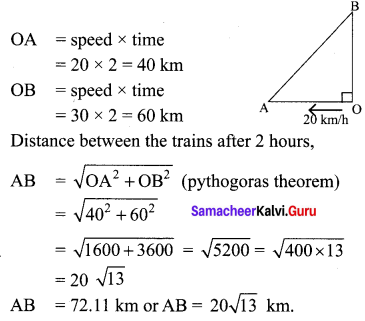Question 6.
D is the mid point of side BC and AE⊥BC. If i BC a, AC = b, AB = c, ED = x, AD = p and AE = h , prove that
(i) b2 = p2 + ax + $$\frac{a^{2}}{4}$$
(ii) c2 = p2 – ax + $$\frac{a^{2}}{4}$$
(iii) b2 + c2 = 2p2 + $$\frac{a^{2}}{2}$$
Solution:
From the figure, D is the mid point of BC.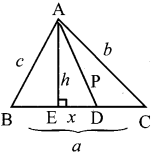We have ∠AED = 90°

AC2 = AD2 + DC2 + 2DC × DE
⇒ AC2 = AD2 + $$\frac{1}{2}$$ BC2 + 2 . $$\frac{1}{2}$$ BC . DE
⇒ AC2 = AD2 + $$\frac{1}{4}$$ BC2 + BC . DE
⇒ AC2 = AD2 + BC . DE + $$\frac{1}{4}$$ BC2
⇒ b2 = p2 + ax + $$\frac{1}{4}$$ a2
Hence proved.

(ii) In ∆ABD, ∠ADE is an acute angle.
AB2 = AD2 + BD2 – 2BD . DE
⇒ AB2 = AD2 + $$\left(\frac{1}{2} \mathrm{BC}\right)^{2}$$ – 2 × $$\frac{1}{2}$$ BC . DE
⇒ AB2 = AD2 + $$\frac{1}{4}$$ BC2 – BC . DE
⇒ AB2 = AD2 – BC . DE + $$\frac{1}{4}$$ BC2
⇒ c2 = p2 – ax + $$\frac{1}{4}$$ a2
Hence proved.

(iii) From (i) and (ii) we get .
AB2 + AC2 = 2AD2 + $$\frac{1}{2}$$ BC2
i.e. c2 + b2 = 2p2 + $$\frac{a^{2}}{2}$$
Hence it is proved.Question 7.
A man whose eye-level is 2 m above the ground wishes to find the height of a tree. He places a mirror horizontally on the ground 20 m from the tree and finds that if he stands at a point C which is 4 m from the mirror B, he can see the reflection of the top of the tree. How height is the tree?
Solution:
From the figure; ∆DAC, ∆FBC are similar triangles and ∆ACE & ∆ABF are similar triangles.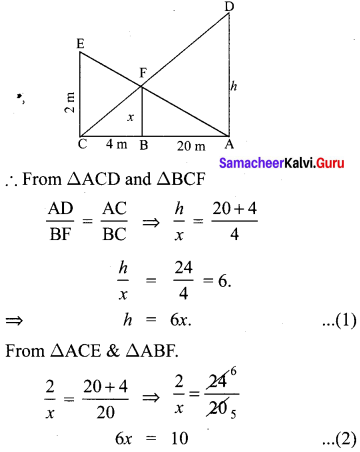∴ height of the tree h = 6x = 10 m.

Question 8.
An emu which is 8 ft tall standing at the foot of a pillar which is 30 ft height. It walks away from the pillar. The shadow of the emu falls beyond emu. What is the relation between the length of the shadow and the distance from the emu to the pillar?
Solution:
Let OA (emu shadow) the x and AB = y.
⇒ pillar’s shadow = OB = OA + AB
⇒ OB = x + y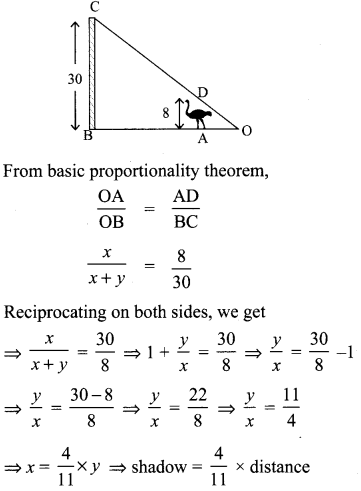Question 9.
Two circles intersect at A and B. From a point P on one of the circles lines PAC and PBD are drawn intersecting the second circle at C and D. Prove that CD is parallel to the tangent at P.
Solution:
Let XY be the tangent at P.
TPT: CD is || to XY.
Construction: Join AB.
∠BAC + ∠BDC= 180° ………… (1)
∠BDC = 180° – ∠BAC …………. (2)
Equating (1) and (2)
we get ∠BDC = ∠PAB
Similarly we get ∠PBA = ∠ACD
as XY is tangent to the circle at ‘P’
∠BPY = ∠PAB (by alternate segment there)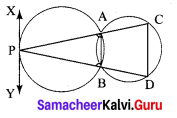∴ ∠PAB = ∠PDC
∠BPY = ∠PDC
XY is parallel of CD.
Hence proved.Question 10.
Let ABC be a triangle and D, E, F are points on the respective sides AB, BC, AC (or their extensions). Let AD: DB = 5 : 3, BE : EC = 3 : 2 and AC = 21. Find the length of the line segment CF.
Solution: